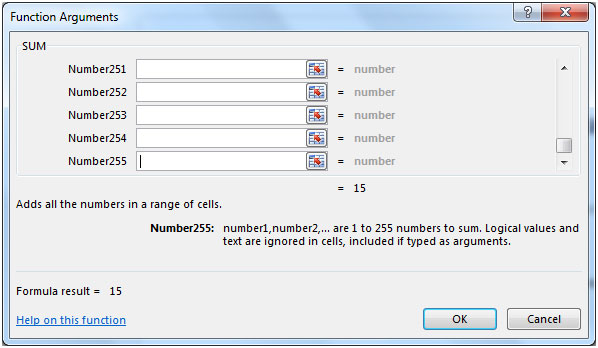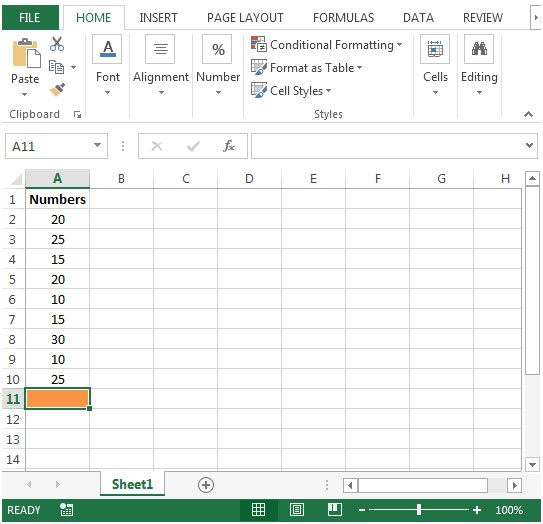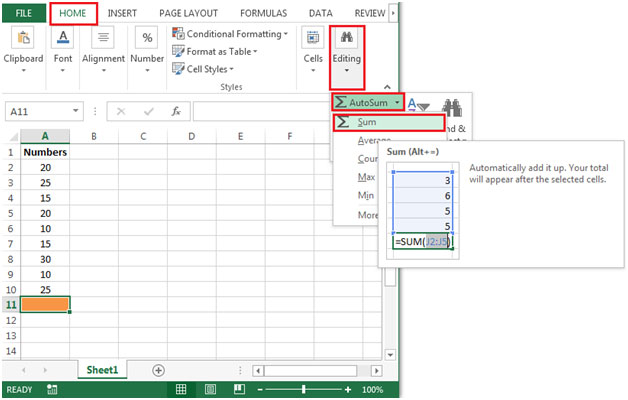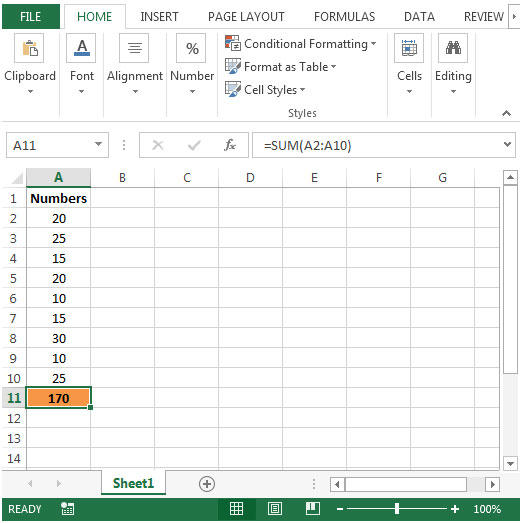# Summing data by selecting cells in Microsoft Excel

To obtain the sum of the data by selecting range of cells, we can use SUM function to get the output.

SUM: Adds all the numbers in a range of cells

Syntax: =SUM(number1,number2,...)

There can be maximum 255 arguments. Refer below shown screenshot:Let us take an example:

To take sum of the numbers in column A.• The formula in cell A11 would be =SUM(A2:A10)
• There is another way for doing sum is by pressing ALT + = or click the Auto Sum feature.

To use Auto Sum feature, you need to follow the below mentioned steps:

• Click on Home ribbon
• In Editing group, click on AutoSum
• From drop down list, select Sum• You will get the sum of the numbers in range A2:A10Terms and Conditions of use

The applications/code on this site are distributed as is and without warranties or liability. In no event shall the owner of the copyrights, or the authors of the applications/code be liable for any loss of profit, any problems or any damage resulting from the use or evaluation of the applications/code.